# Integrals of Motion in T4 Coordinates

In an accompanying Wiki document, we have derived the properties of an orthogonal, axisymmetric, T3 coordinate system in which the first coordinate, λ1, defines a family of concentric oblate-spheroidal surfaces whose (uniform) flattening is defined by a parameter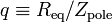$q \equiv R_\mathrm{eq}/Z_\mathrm{pole}$. In a separate, but related, Wiki document, we attempt to derive the 3rd isolating integral of motion for massless particles that move inside a flattened, axisymmetric potential whose equipotential surfaces align with λ1 = constant surfaces in the special (quadratic) case when q2 = 2. While examining this special case, we noticed that, in T3 Coordinates, the h1 and h2 scale factors are only a function of the coordinate ratio λ1 / λ2. This has led us to wonder whether it might be more fruitful to search for the 3rd isolating integral using a coordinate system in which one of the coordinates is defined by this T3-coordinate ratio.

It is with this in mind that we explore the development of a new T4 coordinate system. From the very beginning we will restrict the T4-coordinate definition to the special case of q2 = 2 because, at present, we think that the coordinate T3-coordinate ratio λ1 / λ2 is only interesting in the quadratic case. (See, for example, the polynomial root derived to complete the T1-coordinate inversion for the cubic case q2 = 3; it is another combination of the T3 coordinates that appears to be relevant in the cubic case.)

STOP!

(7/06/2010)

As defined, below, this is not an orthogonal coordinate system.

## Definition

In what follows, the coordinates 123) refer to T3 Coordinates. Let's define a set of orthogonal T4 Coordinates for the special (quadratic) case q2 = 2 such that,

 ξ1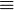$\equiv$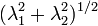$(\lambda_1^2 + \lambda_2^2)^{1/2}$ =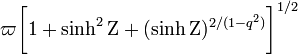$\varpi\biggl[1 + \sinh^2\Zeta + (\sinh\Zeta)^{2/(1-q^2)} \biggr]^{1/2}$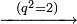$\xrightarrow{~~(q^2=2)~~}$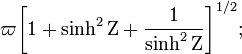$\varpi\biggl[1 + \sinh^2\Zeta + \frac{1}{\sinh^2\Zeta} \biggr]^{1/2} ;$ ξ2$\equiv$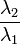$\frac{\lambda_2}{\lambda_1}$ =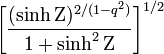$\biggl[ \frac{(\sinh\Zeta)^{2/(1-q^2)}}{1+\sinh^2\Zeta} \biggr]^{1/2}$$\xrightarrow{~~(q^2=2)~~}$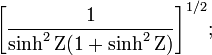$\biggl[ \frac{1}{\sinh^2\Zeta(1+\sinh^2\Zeta)} \biggr]^{1/2} ;$ tanξ3$\equiv$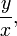$\frac{y}{x} ,$

where,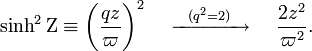$\sinh^2\Zeta \equiv \biggl(\frac{qz}{\varpi}\biggr)^2 ~~~~\xrightarrow{~~(q^2=2)~~} ~~~~ \frac{2z^2}{\varpi^2} .$

The coordinate inversion — from 123) back to 123) — is straightforward. Specifically,

 λ1 =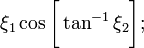$\xi_1 \cos\biggl[ \tan^{-1}\xi_2 \biggr] ;$ λ2 =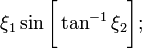$\xi_1 \sin\biggl[ \tan^{-1}\xi_2 \biggr] ;$ λ3 = ξ3.

Here are some relevant partial derivatives: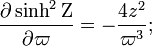$\frac{\partial\sinh^2\Zeta}{\partial\varpi} = -\frac{4z^2}{\varpi^3} ;$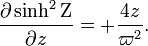$\frac{\partial\sinh^2\Zeta}{\partial z} = + \frac{4z}{\varpi^2} .$

Partial derivatives with respect to cylindrical coordinates are,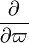$\frac{\partial}{\partial \varpi}$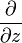$\frac{\partial}{\partial z}$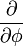$\frac{\partial}{\partial \phi}$ ξ1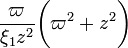$\frac{\varpi}{\xi_1 z^2}\biggl(\varpi^2 + z^2 \biggr)$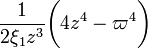$\frac{1}{2\xi_1 z^3}\biggl(4z^4 - \varpi^4 \biggr)$ 0 ξ2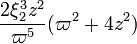$\frac{2\xi_2^3 z^2}{\varpi^5}(\varpi^2 + 4z^2)$$- \frac{2\xi_2^3 z}{\varpi^4}(\varpi^2 + 4z^2)$ 0 ξ3 0 0 1

Hence, the partials with respect to Cartesian coordinates are,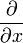$\frac{\partial}{\partial x}$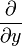$\frac{\partial}{\partial y}$$\frac{\partial}{\partial z}$ ξ1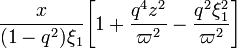$\frac{x}{(1-q^2)\xi_1} \biggl[ 1 + \frac{q^4 z^2}{\varpi^2} - \frac{q^2 \xi_1^2}{\varpi^2} \biggr]$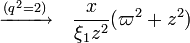$\xrightarrow{(q^2=2)}~~~ \frac{x}{\xi_1 z^2} (\varpi^2 + z^2)$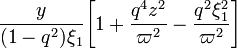$\frac{y}{(1-q^2)\xi_1} \biggl[ 1 + \frac{q^4 z^2}{\varpi^2} - \frac{q^2 \xi_1^2}{\varpi^2} \biggr]$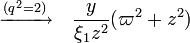$\xrightarrow{(q^2=2)}~~~ \frac{y}{\xi_1 z^2} (\varpi^2 + z^2)$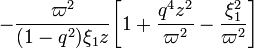$- \frac{\varpi^2}{(1-q^2)\xi_1 z} \biggl[ 1 + \frac{q^4 z^2}{\varpi^2} - \frac{\xi_1^2}{\varpi^2} \biggr]$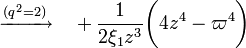$\xrightarrow{(q^2=2)}~~~ + \frac{1}{2\xi_1 z^3}\biggl(4z^4 - \varpi^4 \biggr)$ ξ2$~~$$~~$$~~$ ξ3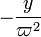$-\frac{y}{\varpi^2}$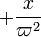$+\frac{x}{\varpi^2}$ 0

The scale factors are,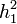$h_1^2$ =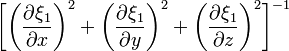$\biggl[ \biggl( \frac{\partial\xi_1}{\partial x} \biggr)^2 + \biggl( \frac{\partial\xi_1}{\partial y} \biggr)^2 + \biggl( \frac{\partial\xi_1}{\partial z} \biggr)^2 \biggr]^{-1}$ =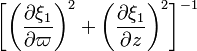$\biggl[ \biggl( \frac{\partial\xi_1}{\partial \varpi} \biggr)^2 + \biggl( \frac{\partial\xi_1}{\partial z} \biggr)^2 \biggr]^{-1}$ =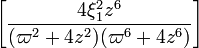$\biggl[ \frac{4\xi_1^2 z^6 }{(\varpi^2 + 4z^2)(\varpi^6 + 4z^6)} \biggr]$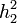$h_2^2$ =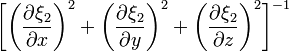$\biggl[ \biggl( \frac{\partial\xi_2}{\partial x} \biggr)^2 + \biggl( \frac{\partial\xi_2}{\partial y} \biggr)^2 + \biggl( \frac{\partial\xi_2}{\partial z} \biggr)^2 \biggr]^{-1}$ =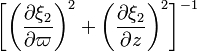$\biggl[ \biggl( \frac{\partial\xi_2}{\partial \varpi} \biggr)^2 + \biggl( \frac{\partial\xi_2}{\partial z} \biggr)^2 \biggr]^{-1}$ =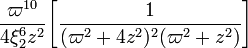$\frac{\varpi^{10}}{4\xi_2^6 z^2} \biggl[ \frac{1}{(\varpi^2 + 4z^2)^2(\varpi^2 + z^2)} \biggr]$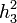$h_3^2$ =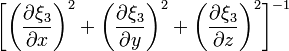$\biggl[ \biggl( \frac{\partial\xi_3}{\partial x} \biggr)^2 + \biggl( \frac{\partial\xi_3}{\partial y} \biggr)^2 + \biggl( \frac{\partial\xi_3}{\partial z} \biggr)^2 \biggr]^{-1}$ =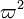$\varpi^2$ where,$~~$.

The position vector is,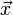$\vec{x}$ =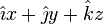$\hat\imath x + \hat\jmath y + \hat{k}z$ =$~~$